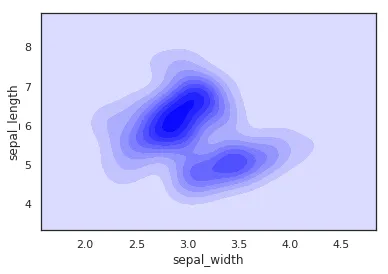## 1.基本密度曲线

    import seaborn as sns
sns.set(color_codes=True)
sns.set_style("white")
sns.kdeplot(df['sepal_width'])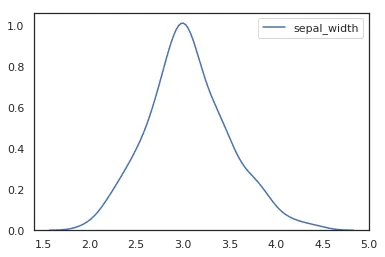## 2.添加阴影的密度曲线

    import seaborn as sns
sns.set(color_codes=True)
sns.set_style("white")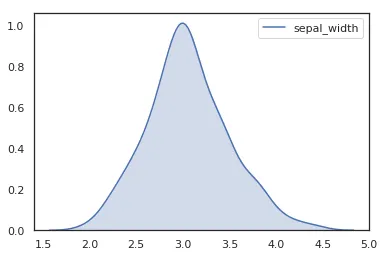## 3.水平方向密度曲线

    import seaborn as sns
sns.set(color_codes=True)
sns.set_style("white")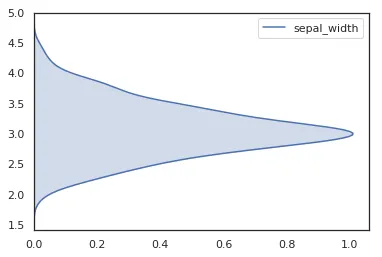vertical指定是否变成横向的密度曲线，虽然英文的意思是垂直的，不是很好理解的样子，但是效果就是变成水平方向。0-0

## 4.频率宽度调整

    import seaborn as sns
sns.set(color_codes=True)
sns.set_style("white")
p1 = sns.kdeplot(df['sepal_width'], shade=True, bw=.5, color="red")
p1 = sns.kdeplot(df['sepal_width'], shade=True, bw=.05, color="blue")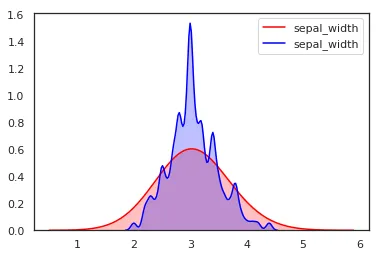## 5.比较多个变量的密度曲线

    import seaborn as sns
sns.set(color_codes=True)
sns.set_style("white")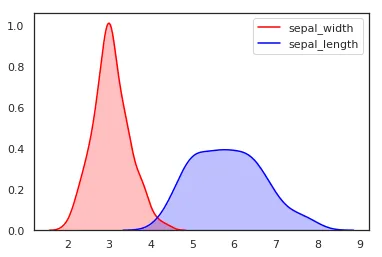## 6.两变量点的密度曲线


import seaborn as sns
sns.set(color_codes=True)
sns.set_style("white")